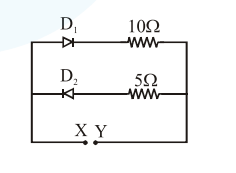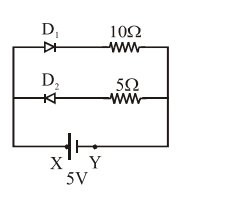# A 5V battery is connected across the points`
Question:

A $5 \mathrm{~V}$ battery is connected across the points $\mathrm{X}$ and $\mathrm{Y}$. Assume $\mathrm{D}_{1}$ and $\mathrm{D}_{2}$ to be normal silicon diodes. Find the current supplied by the battery if the +ve terminal of the battery is connected to point $X$.1. $\sim 0.5 \mathrm{~A}$

2. $\sim 1.5 \mathrm{~A}$

3. $\sim 0.86 \mathrm{~A}$

4. $\sim 0.43 \mathrm{~A}$

Correct Option: , 4

Solution:Here only $D_{1}$ will work and we know for silicon diode, potential drop on $\mathrm{D}_{1}$ will be $0.7 \mathrm{~V}$

$\mathrm{I}=\frac{5-0.7}{10}=0.43 \mathrm{~A}$